Next: Instantaneous frequency Up: HILBERT TRANSFORM Previous: The analytic signal

## Instantaneous envelope

is often used to make the envelope of a signal. The envelope signal can be defined by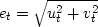.Alternatively, with the analytic signal gt=ut+ivt, the squared envelope is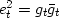.

A quick way to accomplish thephase-shift operation is to use Fourier transformation. Begin with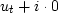, and transform it to the frequency domain. Then multiply by the step function. Finally, inverse transform to get gt=ut+ivt, which is equivalent to.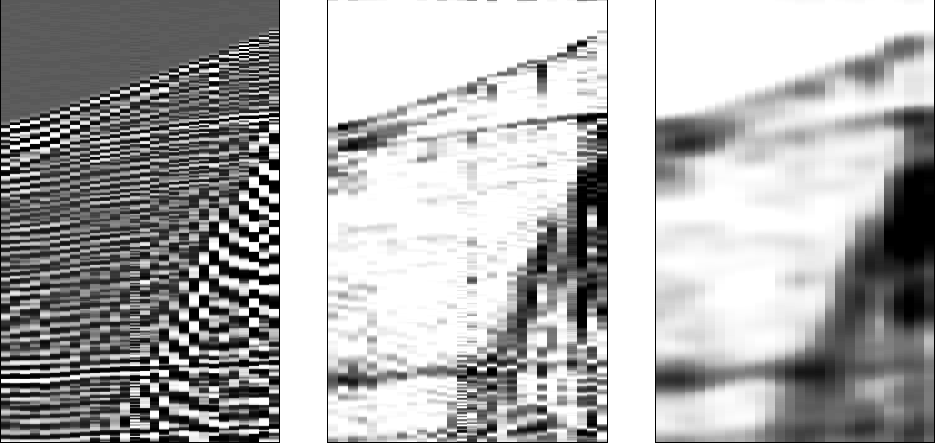envelope
Figure 4
Left is a field profile. Middle is the unsmoothed envelope function. Right is the smoothed envelope. The vertical axis is time and the horizontal axis is space. Independent time-domain calculations are done at each point in space.

Sinusoids have smooth envelope functions, but that does not mean real seismograms do. Figure 4 gives an example of a field profile and unsmoothed and smoothed envelopes. Before smoothing, the stepout (alignment) of the reflections is quite clear. In the practical world, alignment is considered to be a manifestation of phase. An envelope should be a smooth function, such as might be used to scale data without altering its phase. Hence the reason for smoothing the envelope.

If you are interested in wave propagation, you might recognize the possibility of using analytic signals. Energy stored as potential energy isout of phase with kinetic energy, so ut might represent scaled pressure while vt represents scaled velocity. Then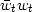is the instantaneous energy. (The scales are the square root of compressibility and the square root of density.)Next: Instantaneous frequency Up: HILBERT TRANSFORM Previous: The analytic signal
Stanford Exploration Project
10/21/1998